# LINEAR FUNCTIONS Graphing Linear Equations LINEAR EQUATIONS LINEARFUNCTION

• Slides: 12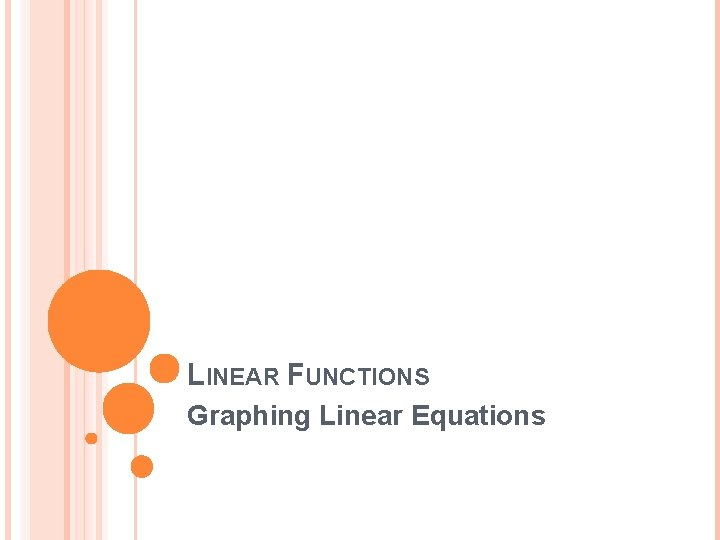LINEAR FUNCTIONS Graphing Linear Equations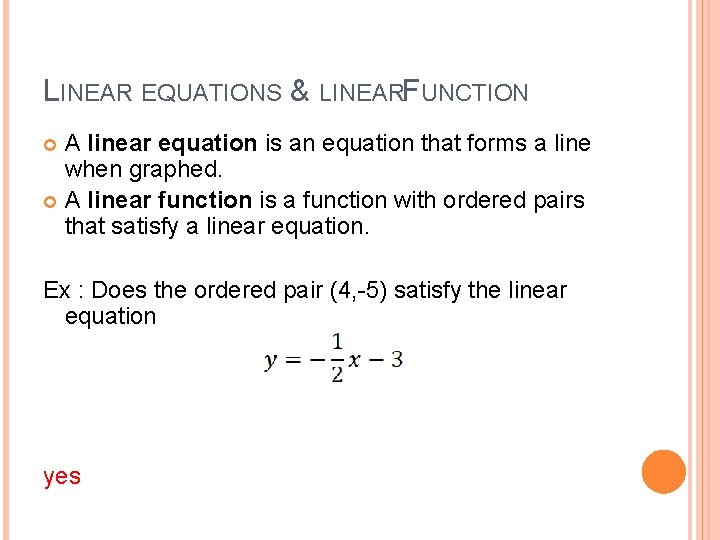LINEAR EQUATIONS & LINEARFUNCTION A linear equation is an equation that forms a line when graphed. A linear function is a function with ordered pairs that satisfy a linear equation. Ex : Does the ordered pair (4, -5) satisfy the linear equation yesFACTS ABOUT LINEAR EQUATIONS No operations other than addition, subtraction, and multiplication of a variable by a constant Variable cannot be found in the denominator Variables cannot be multiplied together Highest exponent on the variable is 1 The graph of a linear equation is a line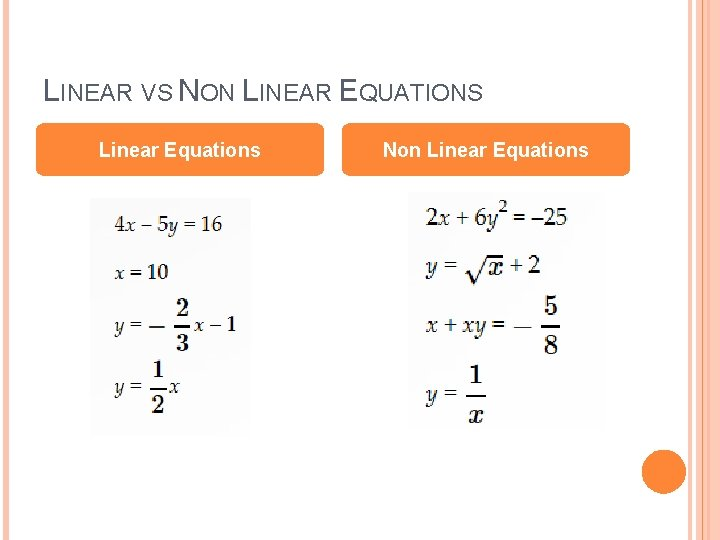LINEAR VS NON LINEAR EQUATIONS Linear Equations Non Linear EquationsSTANDARD FORM OF ALINEAR EQUATION Ax + By = C A, B, C are integers (NO FRACTIONS) (A & B are coefficients, C is a constant) A must be positive No common factorsGRAPHING LINEAR EQUATIONS Method 1: x and y intercepts x-intercept : point where a line intersects the x axis let y = 0 and solve for x Ordered pair: (x, 0) y-intercept: point where a line intersects the y axis let x = 0 and solve for y Ordered pair: (0, y) Method 2: Table of values, y =WRITE EACH EQUATION IN STANDARD FORM. IDENTIFY A, B, AND C. 1) 5 x + 6 y = 3 x + 2 x 2) 3) 5 y +7 = -x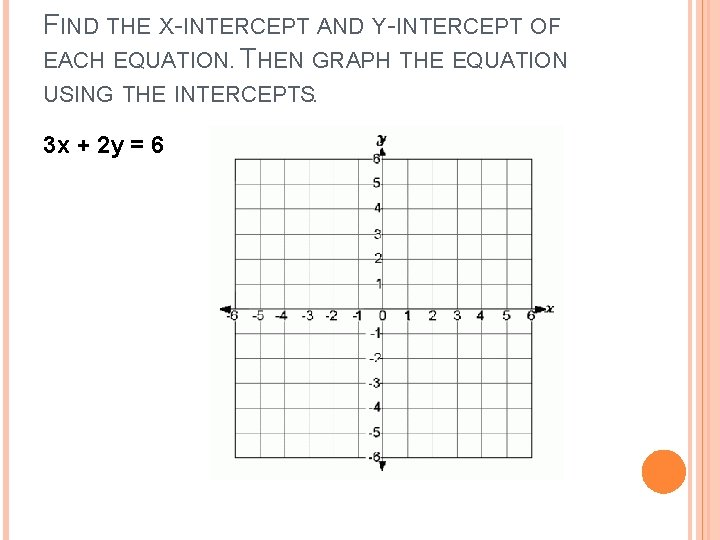FIND THE X-INTERCEPT AND Y-INTERCEPT OF EACH EQUATION. THEN GRAPH THE EQUATION USING THE INTERCEPTS. 3 x + 2 y = 6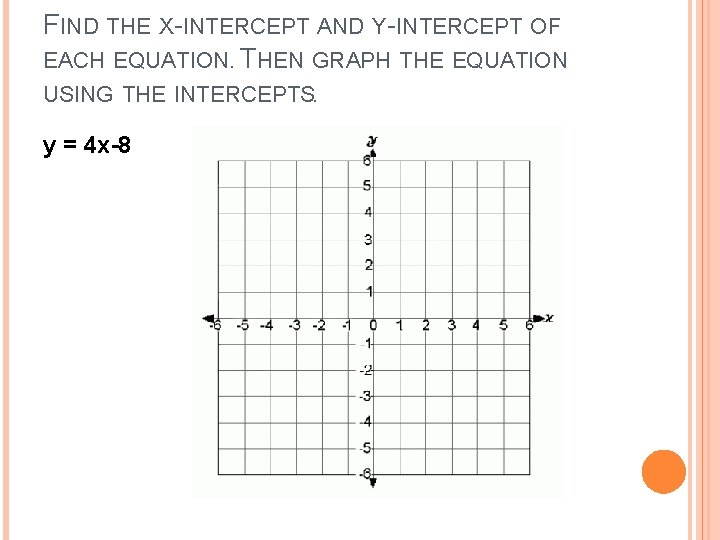FIND THE X-INTERCEPT AND Y-INTERCEPT OF EACH EQUATION. THEN GRAPH THE EQUATION USING THE INTERCEPTS. y = 4 x-8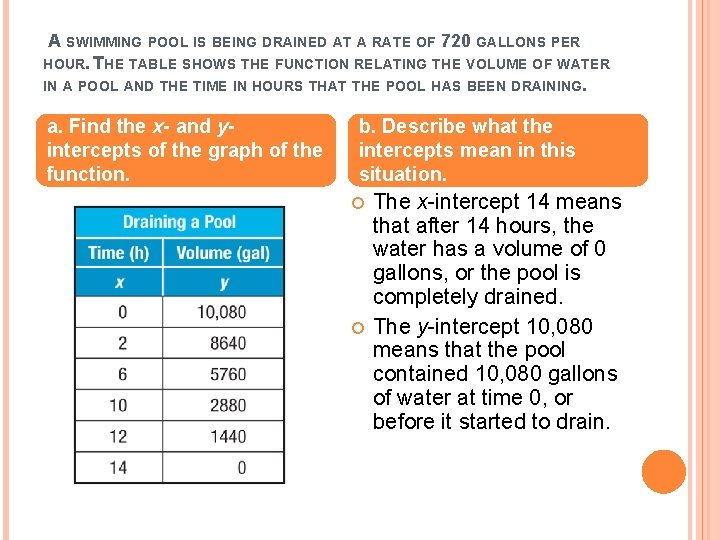A SWIMMING POOL IS BEING DRAINED AT A RATE OF 720 GALLONS PER HOUR. THE TABLE SHOWS THE FUNCTION RELATING THE VOLUME OF WATER IN A POOL AND THE TIME IN HOURS THAT THE POOL HAS BEEN DRAINING. a. Find the x- and yintercepts of the graph of the function. b. Describe what the intercepts mean in this situation. The x-intercept 14 means that after 14 hours, the water has a volume of 0 gallons, or the pool is completely drained. The y-intercept 10, 080 means that the pool contained 10, 080 gallons of water at time 0, or before it started to drain.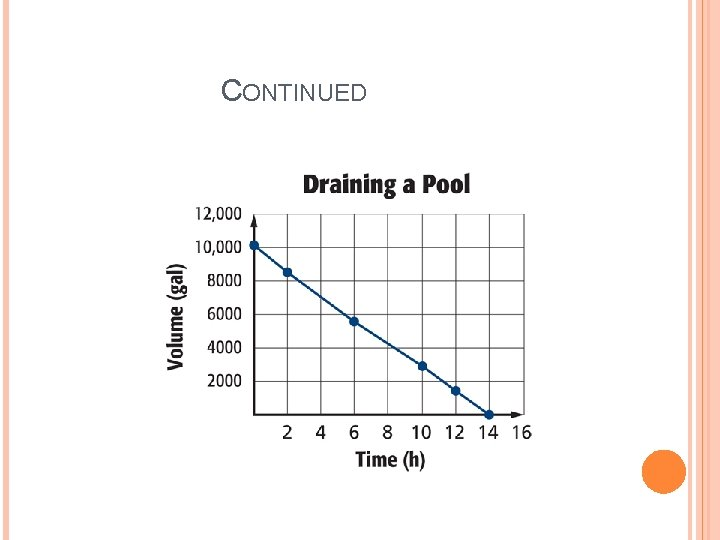CONTINUED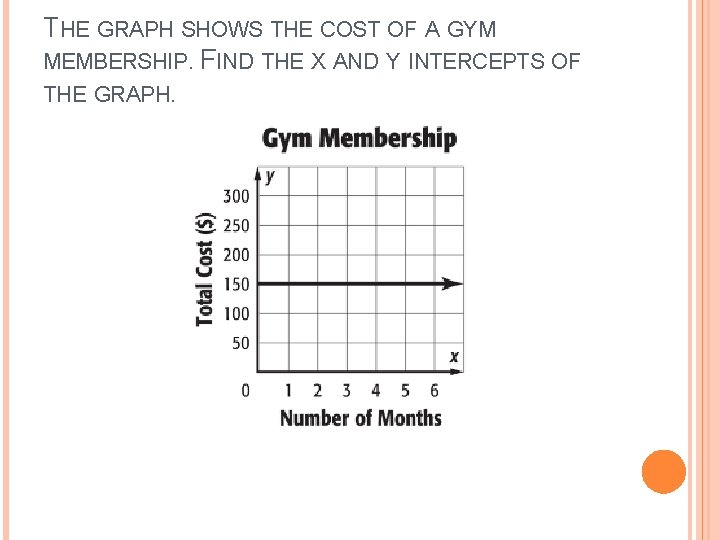THE GRAPH SHOWS THE COST OF A GYM MEMBERSHIP. FIND THE X AND Y INTERCEPTS OF THE GRAPH.